# C++ 缺省参数：学习成为一名优秀的舔狗

• 2023-01-28
河南
• 本文字数：1229 字

阅读完需：约 4 分钟# 一、前言# 二、缺省参数

## 1、缺省参数的定义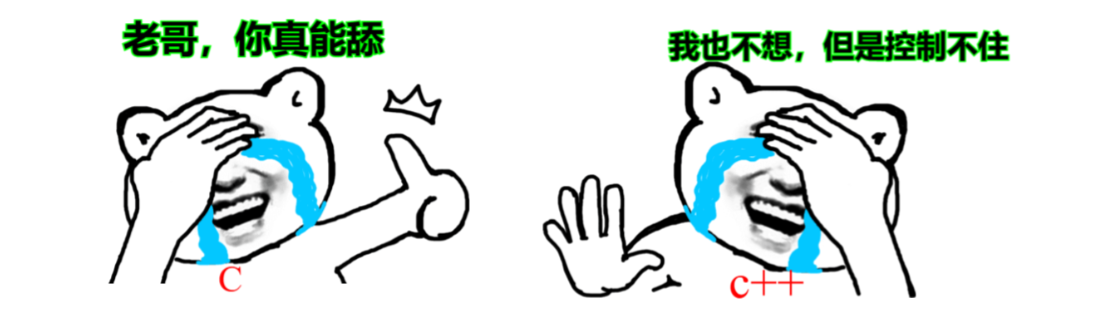void Func(int a = 0){ cout<<a<<endl;}int main(){ Func();      Func(10);   return 0;}

• （1）没有传参时，使用参数的默认值（没有其他人追时，选择舔狗）

• （2）传参时，使用指定的实参（有人追时，忽略舔狗）

## 2、缺省参数的分类

### Ⅰ、全缺省参数void Func(int a = 10, int b = 20, int c = 30) {     cout<<"a = "<<a<<endl;     cout<<"b = "<<b<<endl;     cout<<"c = "<<c<<endl; }

### Ⅱ、半缺省参数

void Func(int a, int b = 10, int c = 20) {     cout<<"a = "<<a<<endl;     cout<<"b = "<<b<<endl;     cout<<"c = "<<c<<endl; }

# 三、注意事项

## 1、直给中间的参数传参

#include<iostream>using namespace std;void Fun(int a = 10, int b = 20, int c = 30){  cout<< a + b + c;}int main(){  Fun(30,,20);  return 0;}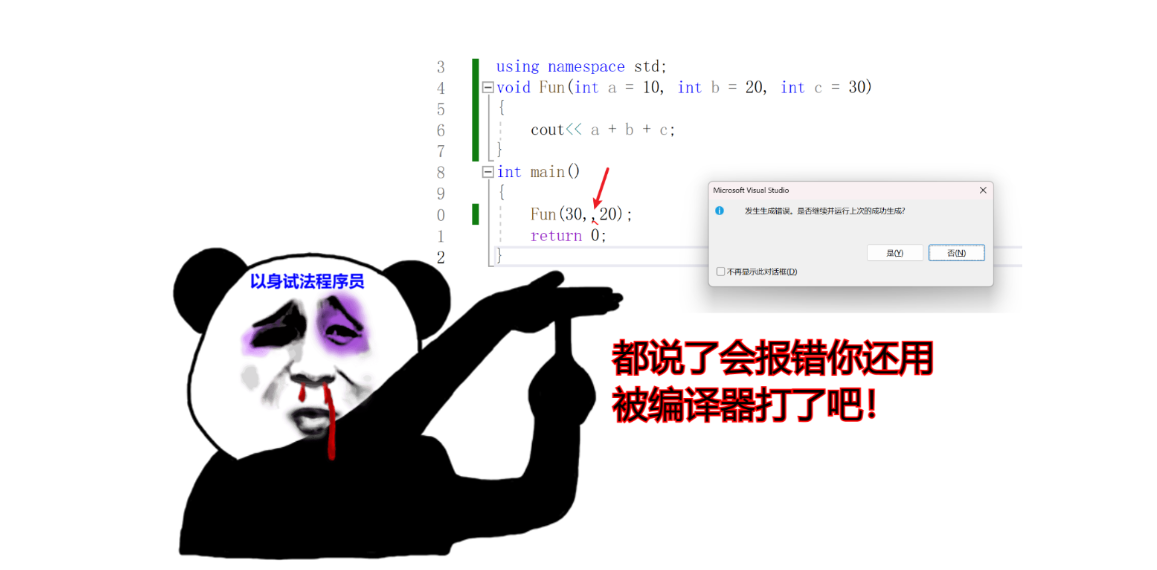## 2、间隔着给缺省参数

void Fun(int a = 10, int b , int c = 30){  cout<< a + b + c;}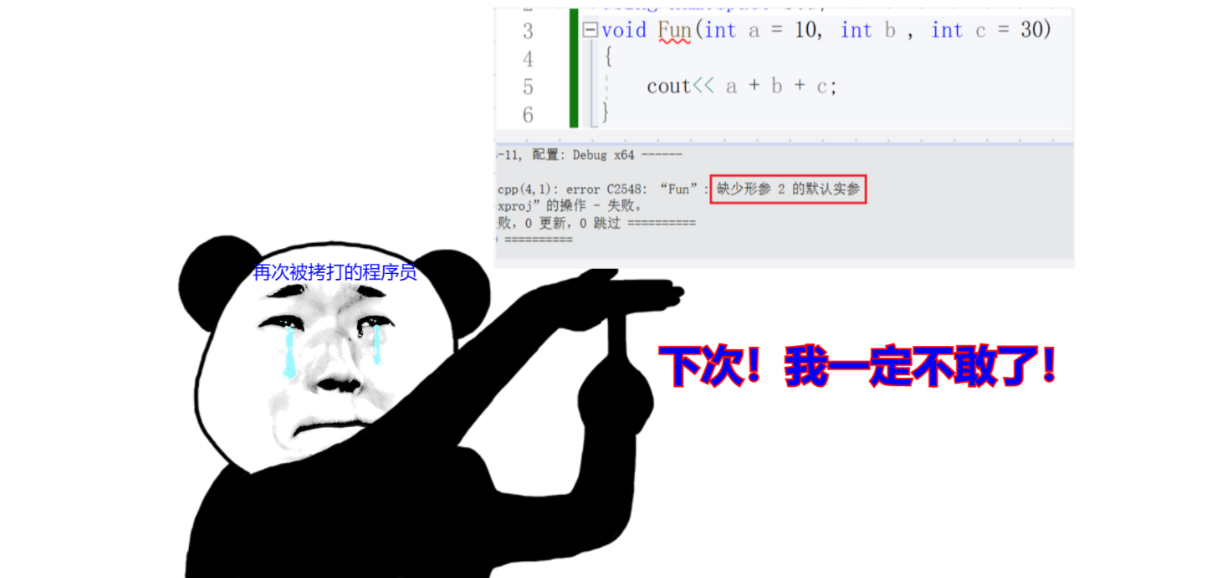void Func(int a, int b = 10, int c = 20) {     cout<<a+b+c; }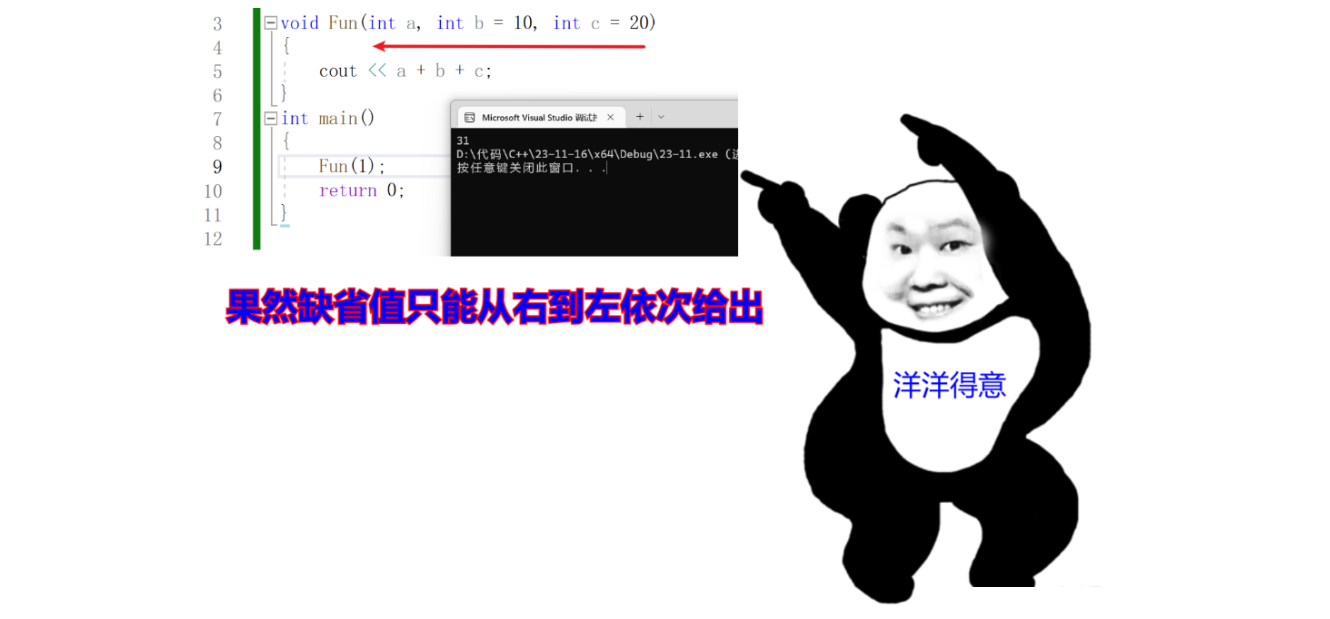## 3、声明和定义中同时出现

  //a.h  void Func(int a = 10);  // a.cpp  void Func(int a = 20) {   //内容 }

# 四、写在后面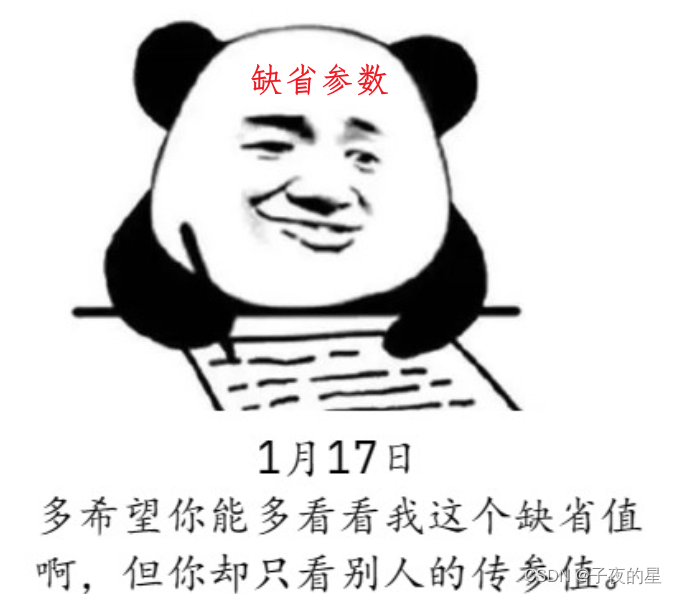## 评论